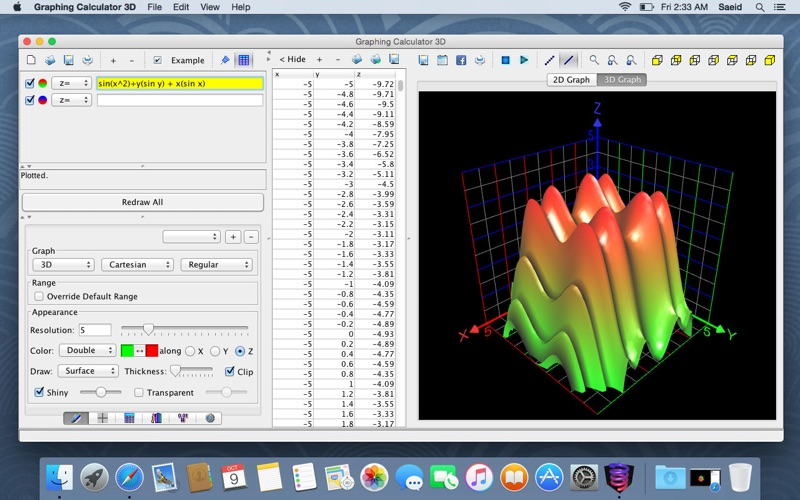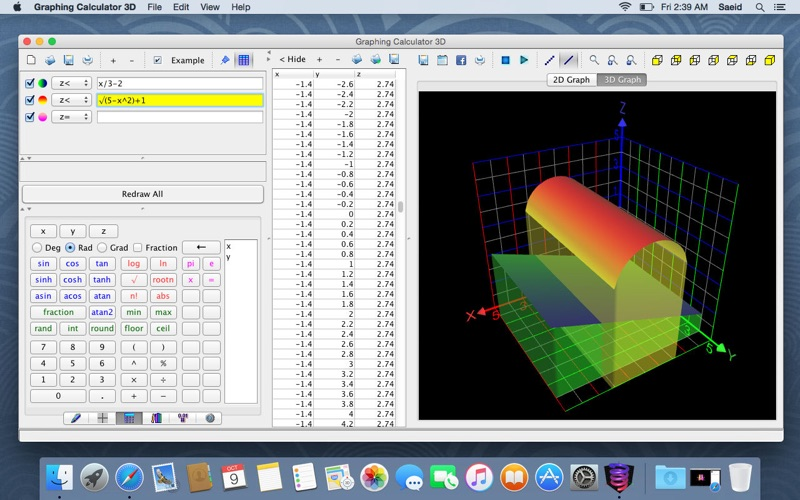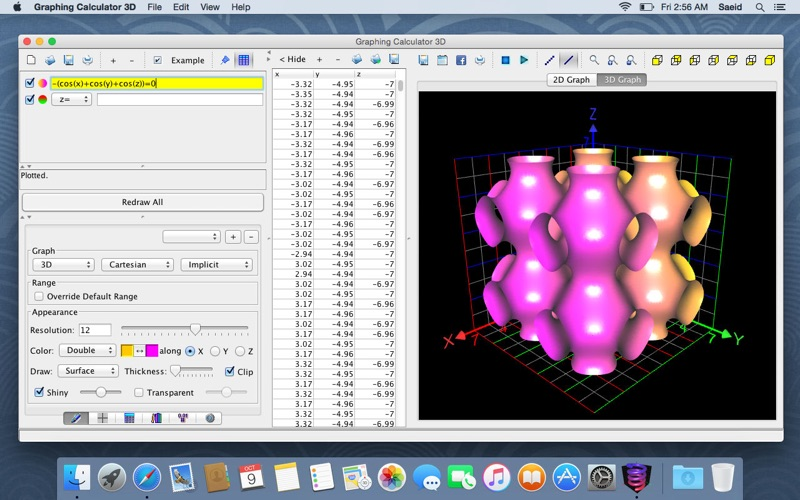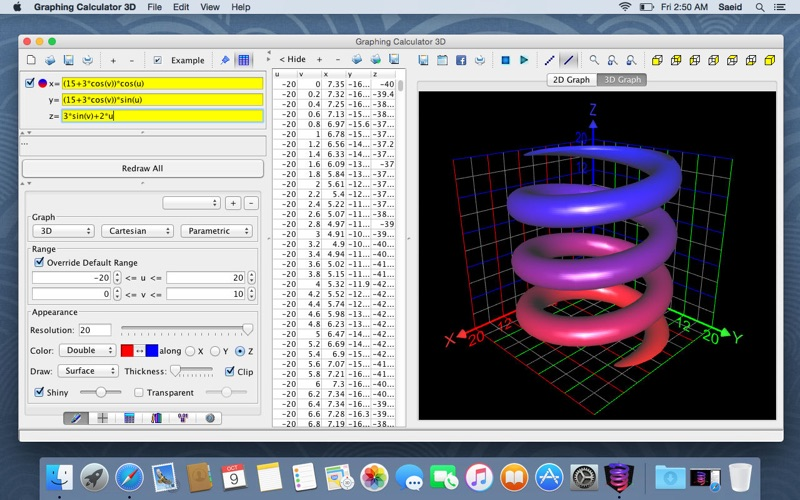# Graphing Calculator 3D

## Mac OSEducation

Free was 4.99

Plot high quality graphs of mathematical equations and data with this easy-to-use software. To plot a function simply type it down and the graph will be updated instantly after each keystroke. Its rich set of features include:

- Plot 2D & 3D functions
- Plot implicit equations
- Plot parametric equations
- Plot inequalities
- Plot 3D scatter points
- Plot contour graphs
- Plot intersections
- Generate tables of values
- Cartesian coordinates
- Polar coordinates
- Cylindrical coordinates
- Spherical coordinates
- Import csv & excel coordinates
- Import live data coordinates
- High precision computations
- 3D shading & glass effects
- Fast rotation & graph animations
- Simultaneous multiple graphs
- Change value of variables via sliders and see their effects on graphs
- Define your own functions (including recursive functions)
- Animate graphs

## What's New

- New modern user-interface
- Smoother 3D graphics with better anti-aliasing
- Faster animation

••••